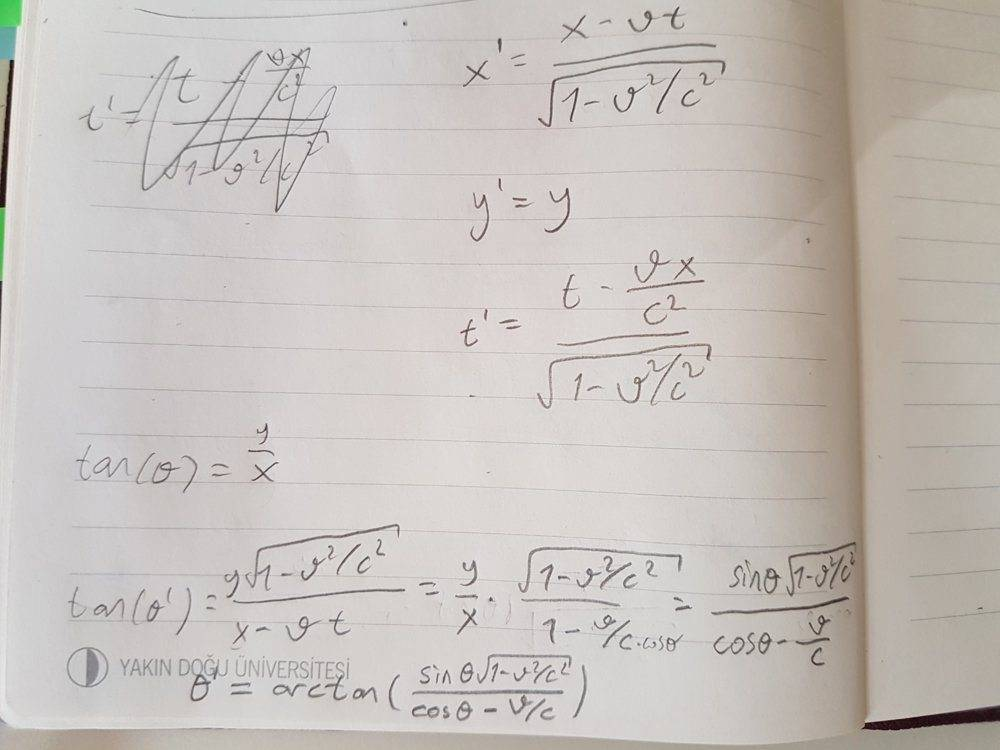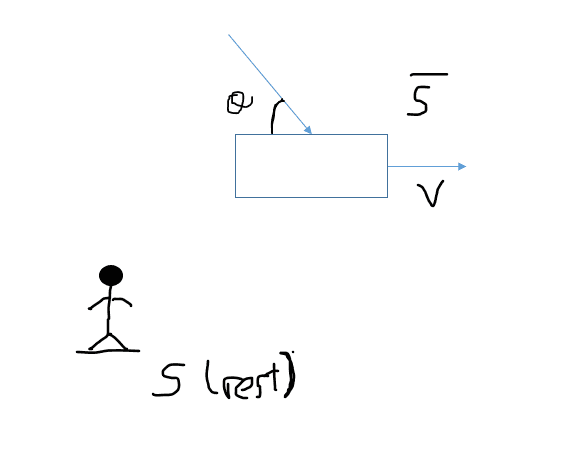# Where's my mistake? (Lorentz Transformation for a moving spaceship)

Homework Statement:
There is a spaceship moving in the +x direction gets a signal from a source on xy plane. From the reference frame of stable stars, the speed of the spaceship is v and the angle that signal creates between x axis and its direction when reached the spaceship is θ. Get help from lorentz transformations and find out the angle θ in the reference frame of spaceship.
Relevant Equations:
x'=(x-vt)/sqrt(1-v^2/c^2)
I didn't use but maybe: t'=(t-(vx)/c^2)/sqrt(1-v^2/c^2)
That's what I found. But the answer is arctan(sinθ*sqrt(1-v^2/c^2)/(cosθ+v/c))Last edited by a moderator:

Rough sketch:$$tan \theta = \frac{y}{x}$$

It is correct but then you don't seem to be using Einstein addition rule correctly. Also note that ##y## and ##x## correspond to the components of the beam's velocity in the ##y## and ##x## directions.

I have labeled the stable star as the rest frame ##S## and the (rectangular) spaceship as the moving frame ##\bar S##.

The key here is noticing that the velocity changes relative to a moving frame not only due to distances (Lorentz contraction) but due to time (time dilation) as well.

The velocity in the ##y## (vertical) direction changes as follows (from ##S## to ##\bar S##):

$$\bar u_y = \frac{d \bar y}{d \bar t} = \frac{d y}{\gamma (dt -v/c^2 dx)} = \frac{u_y}{\gamma (1 -v/c^2 u_x)}$$

Note that there's no Lorentz contraction along ##y## because the spaceship moves along ##x##.

The velocity in the ##x## (horizontal) direction changes as follows (from ##S## to ##\bar S##):

$$\bar u_x = \frac{d \bar x}{d \bar t} = \frac{\gamma(d x - v dt)}{\gamma (dt -v/c^2 dx)} = \frac{u_x -v}{\gamma (1 -v/c^2 u_x)}$$

Knowing that:

$$tan \bar \theta = \frac{\bar u_y}{\bar u_x} = \frac{u_y}{\gamma(u_x -v)}$$

And ##cos\theta = \frac{u_x}{c}## and ##sin\theta = \frac{u_y}{c}##... What do you get? ;)

Please let me know if something is unclear.

Last edited:
Shouldn't dt's are equal?

Typo indeed. Let me fix it.